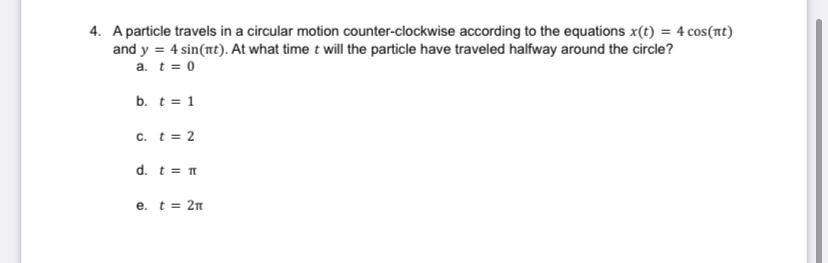Question:

# A particle travels in a circular motion counter-clockwise according to the equations x(t) = 4 cos(πt) and y = 4 sin(πt). At what time will the particle have traveled halfway around the circle? a. t =A particle travels in a circular motion counter-clockwise according to the equations x(t) = 4 cos(πt) and y = 4 sin(πt). At what time will the particle have traveled halfway around the circle? a. t = 0 b. t = 1 C. t = 2 d. t = π e. t = 2π